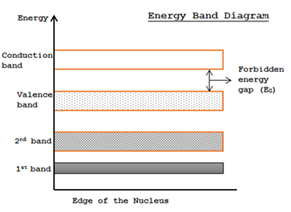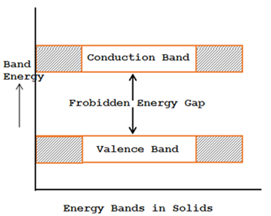# ENERGY BANDS IN SOLIDS

An electron orbiting right close to the nucleus in the 1st shell is very much firmly bound to the nucleus and keeps only a small amount of energy. Therefore initial shell has lowermost energy levels. Larger the distance of an electrons from the nucleus, the better is it energy. From now the energy levels of the outmost shell is maximum. Due to such high energy, valence electrons in the outmost shell can be effortlessly extracted out and therefore such electros takes part in chemical reactions and in bonding with the atoms together. Now this discussion is linked to the electron and shell of one isolated atom merely.In solid, atoms are taken close together. In such an event, outer shell electron is shared by more than one atom. So these electrons come lower than the influence of force from other atom too. The valence electrons are shared by creating a bond through the valence electrons of nearby atoms. Such bonds are termed as covalent bonds. Hence the valence electrons are not free under usual conditions, as they are united by the nearby atoms.

Now the valence electrons hold maximum energy levels. While such electrons forms the covalent bonds, owing to the coupling amongst the valence electrons, the energy levels linked with the valence electrons combine into each other. This inclusion forms an energy band.

In the same way the further energy levels of numerous electrons existent in the 1st orbit, 2nd orbit etc, also combine to form the several energy bands.

So as an alternative of the presence generally separated energy levels as that of the isolated atoms, the closely spread out energy levels are existing in a solid, which are called energy bands.
Out of all the energy bands the important energy bands in the solids are
1. Valance band
2. Conduction band
3. Forbidden energy gap.### Valance band

The range of energies (i.e. band) possessed by valance electrons is known as valance band.
The electrons occupying the outermost orbit or shell of an atom are called valance electrons. In a normal atom; valance bond has the electrons of highest energy. This band may completely or partially filled.

These valence electrons are responsible for forming atomic bonds. The number of valance electrons in an atom also determines the valence of the element whose atom it is. For example, boron is trivalent whereas both Si are Ge are tetravalent i.e., they have four valance electrons each.

### Conduction bond

The range of energies possessed by conduction band electrons is known as conduction band.
The electrons which are responsible for the conduction of current in a conductor are called as conduction electrons.

### Forbidden Energy gap

The separation gap between conduction band and valence band on the energy band diagram is known as forbidden energy gap and measured in the unit of electron-volt (eV).

The width of the forbidden energy gap is a size of the bondage of valence electron to the atom. The larger the energy gap, more closely the valence electron are bound to the nucleus.

In direction to push an electrons from valence band to the conduction band, outer energy equal to the forbidden energy gap must be provided.0 CommentsComments